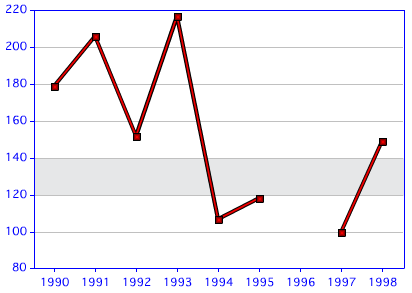The following tip comes from reader Rick Drew. He both asked, and solved this, himself, which makes my job a heck of a lot easier! His question was - how do I create a background 'range' on a chart that can be used to compare against data. In other words, imagine a line chart ranging from 1 to 100. Now imagine that these were golf scores and that the desired score (golfers forgive me, I'm making this up) was 50-70. He wanted to plot a background on the graph for that range so it was really clear to see how the scores compared to the desired value.

Rick found his answer in the chart editor, and in custom XML, which is something I've blogged about numerous times before. Any time you have a question about charts and you can't find the solution in the ColdFusion docs, just pop open the editor and start digging.

Rick found that the limits tag can be used to create a range. Here is a sample:

`<frameChart isInterpolated="false" is3d="false"> <yAxis> <limits index="0" min="120" max="140" color="#e6e7e8"/> </yAxis> </frameChart>`

(Note this XML also turns off interpolation and 3d effects which isn't required for what we are doing.) The limits tag is placed with the yAxis tag. I picked my min and max random based on the data I have for my sample and the color is a gray that matches the rest of the chart. This produces a chart like the one below:Neat trick! I've included a complete script below for folks to play with.

`<cfset q = queryNew("dept,year,sales","varchar,integer,integer")> <!--- generate random sales data ---> <cfloop index="y" from="1990" to="1998"> <cfscript> queryAddRow(q); if(y neq 1996) { querySetCell(q, "dept", "Gamma"); querySetCell(q, "year", y); querySetCell(q, "sales", randRange(80,220)); } else { querySetCell(q, "dept", "Gamma"); querySetCell(q, "year", y); } </cfscript> </cfloop> <cfdump var="#q#">`

`<!--- style from webcharts ---> <cfsavecontent variable="style"> <?xml version="1.0" encoding="UTF-8"?> <frameChart isInterpolated="false" is3d="false"> <yAxis> <limits index="0" min="120" max="140" color="#e6e7e8"/> </yAxis> </frameChart> </cfsavecontent> <p><p> <cfchart chartWidth="400" chartHeight="400" title="Sales" style="#style#"> <cfchartseries type="line" query="q" itemColumn="year" valueColumn="sales" />`

`</cfchart>`

`<cfchart chartWidth="400" chartHeight="400" title="Sales"> <cfchartseries type="line" query="q" itemColumn="year" valueColumn="sales" />`

`</cfchart>`